How to Learn in 24 Hours?The Rapid Learning Movie

 Need Help? M-F: 9am-5pm(PST): Toll-Free: (877) RAPID-10 US Direct: (714) 692-2900 Int'l: 001-714-692-2900 24/7 Online Technical Support: The Rapid Support Center Secure Online Order:Got Questions? Frequently Asked Questions
 Need Proof? Testimonials by Our Users
 Trustlink is a Better Business Bureau Program. Rapid Learning Center is a fivr-star business. External TrustLink Reviews

 Rapid Learning Courses: MCAT in 24 Hours (2015-16) USMLE in 24 Hours (Boards) Chemistry in 24 Hours Biology in 24 Hours Physics in 24 Hours Mathematics in 24 Hours Psychology in 24 Hours SAT in 24 Hours ACT in 24 Hours AP in 24 Hours CLEP in 24 Hours DAT in 24 Hours (Dental) OAT in 24 Hours (Optometry) PCAT in 24 Hours (Pharmacy) Nursing Entrance Exams Certification in 24 Hours eBook - Survival Kits Audiobooks (MP3)

 Tell-A-Friend: Have friends taking science and math courses too? Tell them about our rapid learning system.Home »  Mathematics »  Introductory Statistics

Inference with Proportions and Means

 Topic Review on "Title": Inference: statistical inference is to perform testing and estimation in such a manner as to provide for the evaluation and control over the probabilities of making such errors. Tests of hypothesis: the statement about the values of population parameters may or may not be rejected. Estimation: the process by which sample data are used to indicate the value of an unknown quantity in the population. Experiment: data from a sample are used to test the validity of the hypothesis is called experiment. Statistical hypothesis:  the hypothesis is stated in terms of population parameters such as the mean and variance. Null hypothesis: is a statement about the values of one or more parameters. Alternative hypothesis:  the statement we hope or suspect is true. Type I error: null hypothesis is actually true but the decision is to reject it. Level of significance maximum probability of committing a type I error. Confidence interval: certain probability that the true proportion is within the interval. Tests of significance: are used to assess the evidence provided by data in support of a claim about the population. P-value: the probability that the test statistic would take on as extreme a value as that observed, assuming that the null hypothesis is true. Sample MeanStandard Deviation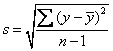Propotion p =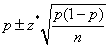Standard Error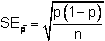Comparing proportionsZ StatisticRapid Study Kit for "Title":
 Flash Movie Flash Game Flash Card Core Concept Tutorial Problem Solving Drill Review Cheat Sheet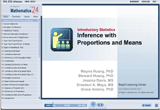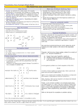"Title" Tutorial Summary : Statistics is the art of decision making in the face of uncertainty. The field of statistical inference consists of those methods used to make decisions or draw conclusions about a population. These methods utilize the information contained in a sample from the population in drawing conclusions. Tests of significance are used to assess the evidence provided by data in support of a claim about the population. The "strength of the evidence" against the claim is used to refute a false claim. Numerical examples are used to illustrate the steps in the hypothesis testing that leads to the final conclusion.

 Tutorial Features: Specific Tutorial Features: The properties of the inference with proportions and means are shown in the examples. Step by step explanation of hypothesis testing is provided. Series Features: Concept map showing inter-connections of new concepts in this tutorial and those previously introduced. Definition slides introduce terms as they are needed. Visual representation of concepts Animated examples—worked out step by step A concise summary is given at the conclusion of the tutorial.

 "Title" Topic List: Inference Test of hypothesis Type I and type II errors Inference on the population mean Confidence interval for proportions One population proportion hypothesis testing Comparing two population proportions

See all 24 lessons in Introductory Statistics, including concept tutorials, problem drills and cheat sheets:
Teach Yourself Introductory Statistics Visually in 24 Hours